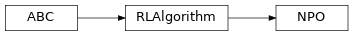# garage.tf.algos.npo¶

class NPO(env_spec, policy, baseline, scope=None, discount=0.99, gae_lambda=1, center_adv=True, positive_adv=False, fixed_horizon=False, pg_loss='surrogate', lr_clip_range=0.01, max_kl_step=0.01, optimizer=None, optimizer_args=None, policy_ent_coeff=0.0, use_softplus_entropy=False, use_neg_logli_entropy=False, stop_entropy_gradient=False, entropy_method='no_entropy', name='NPO')Parameters
• env_spec (EnvSpec) – Environment specification.

• policy (garage.tf.policies.StochasticPolicy) – Policy.

• baseline (garage.tf.baselines.Baseline) – The baseline.

• scope (str) – Scope for identifying the algorithm. Must be specified if running multiple algorithms simultaneously, each using different environments and policies.

• discount (float) – Discount.

• gae_lambda (float) – Lambda used for generalized advantage estimation.

• center_adv (bool) – Whether to rescale the advantages so that they have mean 0 and standard deviation 1.

• positive_adv (bool) – Whether to shift the advantages so that they are always positive. When used in conjunction with center_adv the advantages will be standardized before shifting.

• fixed_horizon (bool) – Whether to fix horizon.

• pg_loss (str) – A string from: ‘vanilla’, ‘surrogate’, ‘surrogate_clip’. The type of loss functions to use.

• lr_clip_range (float) – The limit on the likelihood ratio between policies, as in PPO.

• max_kl_step (float) – The maximum KL divergence between old and new policies, as in TRPO.

• optimizer (object) – The optimizer of the algorithm. Should be the optimizers in garage.tf.optimizers.

• optimizer_args (dict) – The arguments of the optimizer.

• policy_ent_coeff (float) – The coefficient of the policy entropy. Setting it to zero would mean no entropy regularization.

• use_softplus_entropy (bool) – Whether to estimate the softmax distribution of the entropy to prevent the entropy from being negative.

• use_neg_logli_entropy (bool) – Whether to estimate the entropy as the negative log likelihood of the action.

• entropy_method (str) – A string from: ‘max’, ‘regularized’, ‘no_entropy’. The type of entropy method to use. ‘max’ adds the dense entropy to the reward for each time step. ‘regularized’ adds the mean entropy to the surrogate objective. See https://arxiv.org/abs/1805.00909 for more details.

• name (str) – The name of the algorithm.

Note

sane defaults for entropy configuration:
• entropy_method=’max’, center_adv=False, stop_gradient=True (center_adv normalizes the advantages tensor, which will significantly alleviate the effect of entropy. It is also recommended to turn off entropy gradient so that the agent will focus on high-entropy actions instead of increasing the variance of the distribution.)

train(self, trainer)

Obtain samplers and start actual training for each epoch.

Parameters

trainer (Trainer) – Experiment trainer, which rovides services such as snapshotting and sampler control.

Returns

The average return in last epoch cycle.

Return type

float